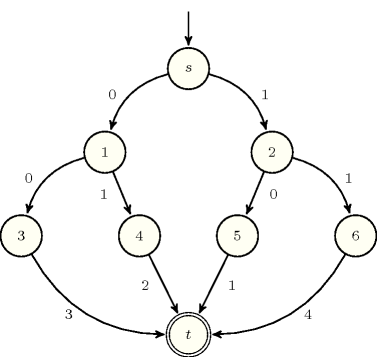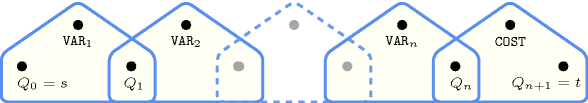## 5.80. cond_lex_cost

Origin

Inspired by [WallaceWilson06].

Constraint

$\mathrm{𝚌𝚘𝚗𝚍}_\mathrm{𝚕𝚎𝚡}_\mathrm{𝚌𝚘𝚜𝚝}\left(\mathrm{𝚅𝙴𝙲𝚃𝙾𝚁},\mathrm{𝙿𝚁𝙴𝙵𝙴𝚁𝙴𝙽𝙲𝙴}_\mathrm{𝚃𝙰𝙱𝙻𝙴},\mathrm{𝙲𝙾𝚂𝚃}\right)$

Type
 $\mathrm{𝚃𝚄𝙿𝙻𝙴}_\mathrm{𝙾𝙵}_\mathrm{𝚅𝙰𝙻𝚂}$ $\mathrm{𝚌𝚘𝚕𝚕𝚎𝚌𝚝𝚒𝚘𝚗}\left(\mathrm{𝚟𝚊𝚕}-\mathrm{𝚒𝚗𝚝}\right)$
Arguments
 $\mathrm{𝚅𝙴𝙲𝚃𝙾𝚁}$ $\mathrm{𝚌𝚘𝚕𝚕𝚎𝚌𝚝𝚒𝚘𝚗}\left(\mathrm{𝚟𝚊𝚛}-\mathrm{𝚍𝚟𝚊𝚛}\right)$ $\mathrm{𝙿𝚁𝙴𝙵𝙴𝚁𝙴𝙽𝙲𝙴}_\mathrm{𝚃𝙰𝙱𝙻𝙴}$ $\mathrm{𝚌𝚘𝚕𝚕𝚎𝚌𝚝𝚒𝚘𝚗}\left(\mathrm{𝚝𝚞𝚙𝚕𝚎}-\mathrm{𝚃𝚄𝙿𝙻𝙴}_\mathrm{𝙾𝙵}_\mathrm{𝚅𝙰𝙻𝚂}\right)$ $\mathrm{𝙲𝙾𝚂𝚃}$ $\mathrm{𝚍𝚟𝚊𝚛}$
Restrictions
 $|\mathrm{𝚃𝚄𝙿𝙻𝙴}_\mathrm{𝙾𝙵}_\mathrm{𝚅𝙰𝙻𝚂}|\ge 1$ $\mathrm{𝚛𝚎𝚚𝚞𝚒𝚛𝚎𝚍}$$\left(\mathrm{𝚃𝚄𝙿𝙻𝙴}_\mathrm{𝙾𝙵}_\mathrm{𝚅𝙰𝙻𝚂},\mathrm{𝚟𝚊𝚕}\right)$ $\mathrm{𝚛𝚎𝚚𝚞𝚒𝚛𝚎𝚍}$$\left(\mathrm{𝚅𝙴𝙲𝚃𝙾𝚁},\mathrm{𝚟𝚊𝚛}\right)$ $|\mathrm{𝚅𝙴𝙲𝚃𝙾𝚁}|=|\mathrm{𝚃𝚄𝙿𝙻𝙴}_\mathrm{𝙾𝙵}_\mathrm{𝚅𝙰𝙻𝚂}|$ $\mathrm{𝚛𝚎𝚚𝚞𝚒𝚛𝚎𝚍}$$\left(\mathrm{𝙿𝚁𝙴𝙵𝙴𝚁𝙴𝙽𝙲𝙴}_\mathrm{𝚃𝙰𝙱𝙻𝙴},\mathrm{𝚝𝚞𝚙𝚕𝚎}\right)$ $\mathrm{𝚜𝚊𝚖𝚎}_\mathrm{𝚜𝚒𝚣𝚎}$$\left(\mathrm{𝙿𝚁𝙴𝙵𝙴𝚁𝙴𝙽𝙲𝙴}_\mathrm{𝚃𝙰𝙱𝙻𝙴},\mathrm{𝚝𝚞𝚙𝚕𝚎}\right)$ $\mathrm{𝚍𝚒𝚜𝚝𝚒𝚗𝚌𝚝}$$\left(\mathrm{𝙿𝚁𝙴𝙵𝙴𝚁𝙴𝙽𝙲𝙴}_\mathrm{𝚃𝙰𝙱𝙻𝙴},\left[\right]\right)$ $\mathrm{𝚒𝚗}_\mathrm{𝚛𝚎𝚕𝚊𝚝𝚒𝚘𝚗}$$\left(\mathrm{𝚅𝙴𝙲𝚃𝙾𝚁},\mathrm{𝙿𝚁𝙴𝙵𝙴𝚁𝙴𝙽𝙲𝙴}_\mathrm{𝚃𝙰𝙱𝙻𝙴}\right)$ $\mathrm{𝙲𝙾𝚂𝚃}\ge 1$ $\mathrm{𝙲𝙾𝚂𝚃}\le |\mathrm{𝙿𝚁𝙴𝙵𝙴𝚁𝙴𝙽𝙲𝙴}_\mathrm{𝚃𝙰𝙱𝙻𝙴}|$
Purpose

$\mathrm{𝚅𝙴𝙲𝚃𝙾𝚁}$ is assigned to the ${\mathrm{𝙲𝙾𝚂𝚃}}^{th}$ item of the collection $\mathrm{𝙿𝚁𝙴𝙵𝙴𝚁𝙴𝙽𝙲𝙴}_\mathrm{𝚃𝙰𝙱𝙻𝙴}$.

Example
$\left(\begin{array}{c}〈0,1〉,\hfill \\ 〈\begin{array}{c}\mathrm{𝚝𝚞𝚙𝚕𝚎}-〈1,0〉,\hfill \\ \mathrm{𝚝𝚞𝚙𝚕𝚎}-〈0,1〉,\hfill \\ \mathrm{𝚝𝚞𝚙𝚕𝚎}-〈0,0〉,\hfill \\ \mathrm{𝚝𝚞𝚙𝚕𝚎}-〈1,1〉\hfill \end{array}〉,2\hfill \end{array}\right)$

The $\mathrm{𝚌𝚘𝚗𝚍}_\mathrm{𝚕𝚎𝚡}_\mathrm{𝚌𝚘𝚜𝚝}$ constraint holds since $\mathrm{𝚅𝙴𝙲𝚃𝙾𝚁}$ is assigned to the second item of the collection $\mathrm{𝙿𝚁𝙴𝙵𝙴𝚁𝙴𝙽𝙲𝙴}_\mathrm{𝚃𝙰𝙱𝙻𝙴}$.

Typical
 $|\mathrm{𝚃𝚄𝙿𝙻𝙴}_\mathrm{𝙾𝙵}_\mathrm{𝚅𝙰𝙻𝚂}|>1$ $|\mathrm{𝚅𝙴𝙲𝚃𝙾𝚁}|>1$ $|\mathrm{𝙿𝚁𝙴𝙵𝙴𝚁𝙴𝙽𝙲𝙴}_\mathrm{𝚃𝙰𝙱𝙻𝙴}|>1$
Symmetries
• Items of $\mathrm{𝚅𝙴𝙲𝚃𝙾𝚁}$ and $\mathrm{𝙿𝚁𝙴𝙵𝙴𝚁𝙴𝙽𝙲𝙴}_\mathrm{𝚃𝙰𝙱𝙻𝙴}.\mathrm{𝚝𝚞𝚙𝚕𝚎}$ are permutable (same permutation used).

• All occurrences of two distinct tuples of values in $\mathrm{𝚅𝙴𝙲𝚃𝙾𝚁}$ or $\mathrm{𝙿𝚁𝙴𝙵𝙴𝚁𝙴𝙽𝙲𝙴}_\mathrm{𝚃𝙰𝙱𝙻𝙴}.\mathrm{𝚝𝚞𝚙𝚕𝚎}$ can be swapped; all occurrences of a tuple of values in $\mathrm{𝚅𝙴𝙲𝚃𝙾𝚁}$ or $\mathrm{𝙿𝚁𝙴𝙵𝙴𝚁𝙴𝙽𝙲𝙴}_\mathrm{𝚃𝙰𝙱𝙻𝙴}.\mathrm{𝚝𝚞𝚙𝚕𝚎}$ can be renamed to any unused tuple of values.

Usage

We consider an example taken from [WallaceWilson06] were a customer has to decide among vacations. There are two seasons when he can travel ($\mathrm{𝚜𝚙𝚛𝚒𝚗𝚐}$ and $\mathrm{𝚜𝚞𝚖𝚖𝚎𝚛}$) and two locations $\mathrm{𝙽𝚊𝚙𝚕𝚎𝚜}$ and $\mathrm{𝙷𝚎𝚕𝚜𝚒𝚗𝚔𝚒}$. Furthermore assume that location is more important than season and the preferred period of the year depends on the selected location. The travel preferences of a customer are explicitly defined by stating the preferences ordering among the possible tuples of values $〈\mathrm{𝙽𝚊𝚙𝚕𝚎𝚜},\mathrm{𝚜𝚙𝚛𝚒𝚗𝚐}〉$, $〈\mathrm{𝙽𝚊𝚙𝚕𝚎𝚜},\mathrm{𝚜𝚞𝚖𝚖𝚎𝚛}〉$, $〈\mathrm{𝙷𝚎𝚕𝚜𝚒𝚗𝚔𝚒},\mathrm{𝚜𝚙𝚛𝚒𝚗𝚐}〉$ and $〈\mathrm{𝙷𝚎𝚕𝚜𝚒𝚗𝚔𝚒},\mathrm{𝚜𝚞𝚖𝚖𝚎𝚛}〉$. For instance we may state within the preference table $\mathrm{𝙿𝚁𝙴𝙵𝙴𝚁𝙴𝙽𝙲𝙴}_\mathrm{𝚃𝙰𝙱𝙻𝙴}$ of the $\mathrm{𝚌𝚘𝚗𝚍}_\mathrm{𝚕𝚎𝚡}_\mathrm{𝚌𝚘𝚜𝚝}$ constraint the preference ordering $〈\mathrm{𝙽𝚊𝚙𝚕𝚎𝚜},\mathrm{𝚜𝚙𝚛𝚒𝚗𝚐}〉\succ 〈\mathrm{𝙷𝚎𝚕𝚜𝚒𝚗𝚔𝚒},\mathrm{𝚜𝚞𝚖𝚖𝚎𝚛}〉\succ 〈\mathrm{𝙷𝚎𝚕𝚜𝚒𝚗𝚔𝚒},\mathrm{𝚜𝚙𝚛𝚒𝚗𝚐}〉\succ 〈\mathrm{𝙽𝚊𝚙𝚕𝚎𝚜},\mathrm{𝚜𝚞𝚖𝚖𝚎𝚛}〉$, which denotes the fact that our customer prefers $\mathrm{𝙽𝚊𝚙𝚕𝚎𝚜}$ in the $\mathrm{𝚜𝚙𝚛𝚒𝚗𝚐}$ and $\mathrm{𝙷𝚎𝚕𝚜𝚒𝚗𝚔𝚒}$ in the $\mathrm{𝚜𝚞𝚖𝚖𝚎𝚛}$, and a vacation in $\mathrm{𝚜𝚙𝚛𝚒𝚗𝚐}$ is preferred over $\mathrm{𝚜𝚞𝚖𝚖𝚎𝚛}$. Finally a solution minimising the cost variable $\mathrm{𝙲𝙾𝚂𝚃}$ will match the preferences stated by our customer.

attached to cost variant: $\mathrm{𝚒𝚗}_\mathrm{𝚛𝚎𝚕𝚊𝚝𝚒𝚘𝚗}$ ($\mathrm{𝙲𝙾𝚂𝚃}$ parameter removed).

specialisation: $\mathrm{𝚎𝚕𝚎𝚖𝚎𝚗𝚝}$ ($\mathrm{𝚝𝚞𝚙𝚕𝚎}$ of $\mathrm{𝚟𝚊𝚛𝚒𝚊𝚋𝚕𝚎𝚜}$ replaced by single $\mathrm{𝚟𝚊𝚛𝚒𝚊𝚋𝚕𝚎}$).

Keywords
Automaton

Figure 5.80.1 depicts the automaton associated with $\mathrm{𝚌𝚘𝚗𝚍}_\mathrm{𝚕𝚎𝚡}_\mathrm{𝚕𝚎𝚜𝚜𝚎𝚚}$ constraint. Let ${\mathrm{𝚅𝙰𝚁}}_{k}$ denote the $\mathrm{𝚟𝚊𝚛}$ attribute of the ${k}^{th}$ item of the $\mathrm{𝚅𝙴𝙲𝚃𝙾𝚁}$ collection. Figure 5.80.2 depicts the reformulation of the $\mathrm{𝚌𝚘𝚗𝚍}_\mathrm{𝚕𝚎𝚡}_\mathrm{𝚌𝚘𝚜𝚝}$ constraint.

##### Figure 5.80.1. Automaton of the $\mathrm{𝚌𝚘𝚗𝚍}_\mathrm{𝚕𝚎𝚡}_\mathrm{𝚌𝚘𝚜𝚝}$ constraint given in the Example slot##### Figure 5.80.2. Hypergraph of the reformulation corresponding to the automaton of the $\mathrm{𝚌𝚘𝚗𝚍}_\mathrm{𝚕𝚎𝚡}_\mathrm{𝚌𝚘𝚜𝚝}$ constraint